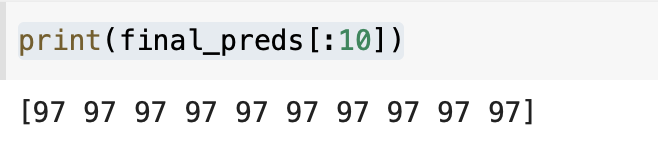# How I can create a prediction on Pytorch LSTM?

I am building a multi label classifier for text using LSTM. I am using this snippet of code to train the model. How I can create a prediction on my test data to send it for submission:

import torch.nn.functional as F
train_loss = 0
val_loss = 0
for epoch in range(1, epochs + 1):
model.train()
batch_X, batch_y = batch

``````        batch_X = batch_X.to(device)
batch_y = batch_y.to(device)
# batch_X = F.sigmoid(batch_X)
batch_X= F.relu(batch_X)

#batch_len = batch_X.t()[0,:]
#print(batch_len)

# TODO: Complete this train method to train the model provided.
output = model.forward(batch_X)
# print(output)

#_, batch_y = batch_y.max(dim=1)
# batch_y = batch_y.reshape(-1,1)

# output = output.view(output.size(0), batch_y.size(1))

loss = loss_fn(output, batch_y)
loss.backward()
optimizer.step()

train_loss += loss.item()
#print(loss.item())
#break
model.eval()
batch_X, batch_y = batch

batch_X = batch_X.to(device)
batch_y = batch_y.to(device)

# TODO: Complete this train method to train the model provided.
output = model.forward(batch_X)

#_, batch_y = batch_y.max(dim=1)
# batch_y = batch_y.reshape(-1,1)
#print(output)

loss = loss_fn(output, batch_y)
val_loss += loss.item()
#break

print("Epoch: {}, BCE Train Loss: {} Valid Loss {}".format(epoch, total_train_loss, total_val_loss))
val_loss = 0
train_loss = 0
#break

return model
``````

model = LSTMClassifier(embedding_dim, hidden_dim, vocab_size).to(device)
loss_fn = torch.nn.BCELoss()

model_tn = train(model, train_sample_dl, var_sample_dl, num_epochs, optimizer, loss_fn, device)

You shouldn’t b using relu here.

this shouldn’t be required.

Instead use `output = model(batch_X)`

This may not be required.

You might need `torch.nn.BCEWithLogitsLoss()` depending on what the output of your `model` is like.

Thank you so much, I reconstruct the code as you recommended. now I have this issue. any suggestions.

ValueError: y contains previously unseen labels: [0.5 0.50008935]

need to have the return as the label indices like thisThis is not related to your model or Pytorch, this is sklearn error.
Are you referring to some codebase?